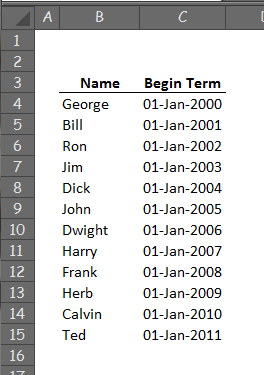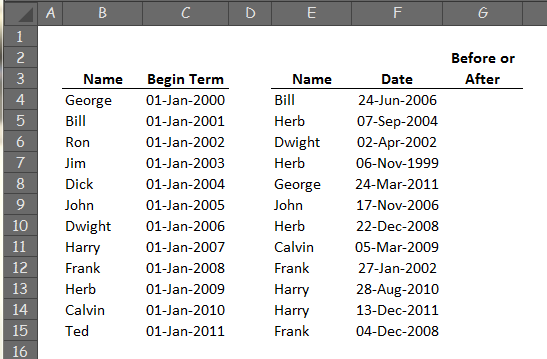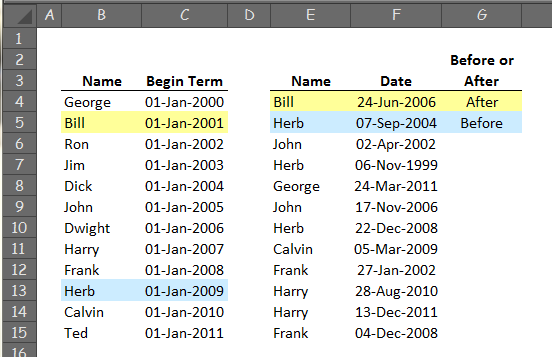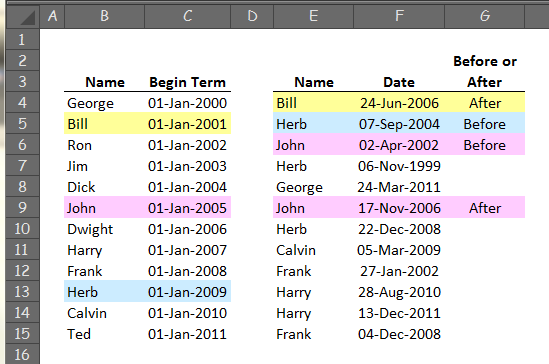Excel =LOOKUP() function with two variableson
Hello,

Suppose the 12 individuals, whose names are shown in the following figure (Fig. 1), have each served as the President of a certain organization.Suppose also that each president served for a term of one calendar year and the dates in column C indicate the date on which each respective person began their term.

Next, suppose that column F in Fig. 2 shows the dates of some arbitrary event involving a single individual whose name is shown in column E.What formula in column G would determine whether the event for a particular person, occurred Before or After the first day of that person's term as President?

For example, the event for Bill occurred on 24-Jun-2006 (Fig. 3) which was After 01-Jan-2001, the beginning of his term. In contrast, Herb's event on 07-Sep-2004 took place Before the first day of his term on 01-Jan-2009.Another example, shown in Fig. 4, indicates that John had two events.  The first, on 02-Apr-2002, took place Before his first day as President (01-Jan-2005), while the second, on 17-Nov-2006, took place After.So, as stated above, my question is:

What formula in column G would give these Before's and After's automatically?  I think there is a =VLOOKUP() in there somewhere but I am not sure how to put it together.

Thanks
Comment
Watch Question

Do more withEXPERT OFFICE® is a registered trademark of EXPERTS EXCHANGE®
Most Valuable Expert 2013
Commented:
Hello Steve, in G4 try this formula copied down

=IF(F4<VLOOKUP(E4,B\$4:C\$15,2,0),"Before","After")

...if the name doesn't exist in the list you'll get #N/A error so you can add IFERROR and use this version to prevent that

=IFERROR(IF(F4<VLOOKUP(E4,B\$4:C\$15,2,0),"Before","After"),"Name not in list")

regards, barry

Commented:
Thanks Barry.  That works great!

I've got a question about the last argument (range_lookup) in VLOOKUP.  (I'm including the syntax and description below,only for my own future reference.)

Reading the description seems to indicate that the main purpose of range_lookup is to specify whether close-approximation matches  are allowed or whether the match must be exact.  Is that correct?

Also, it talks about the range_lookup being either TRUE or FALSE or omitted.  I understand how an argument can be omitted but I don't understand how it can be TRUE or FALSE.  Those terms usually apply to the result. Furthermore, I don't understand the "0" value included in your solution.

Thanks again.

^^^^^^^^^^^^^^^^^^^^^^^^^^^^^^

Syntax:
VLOOKUP(lookup_value, table_array, col_index_num, [range_lookup])

Description:
range_lookup  Optional. A logical value that specifies whether you want VLOOKUP to find an exact match or an approximate match:

If range_lookup is either TRUE or is omitted, an exact or approximate match is returned. If an exact match is not found, the next largest value that is less than lookup_value is returned.

Important  If range_lookup is either TRUE or is omitted, the values in the first column of table_array must be placed in ascending sort order; otherwise, VLOOKUP might not return the correct value.

For more information, see Sort data in a range or table.

If range_lookup is FALSE, the values in the first column of table_array do not need to be sorted.

If the range_lookup argument is FALSE, VLOOKUP will find only an exact match. If there are two or more values in the first column of table_array that match the lookup_value, the first value found is used. If an exact match is not found, the error value #N/A is returned.

Commented:
NO POINTS FOR THIS

Yes this is correct. Except that I am not comfortable with the word "approximate" used in the help file. The value result of the search might be closer to the next higher value but it will return the next lower value if exact not found.

If omitted then the default value is "true".

Most Valuable Expert 2013

Commented:
Hello Steve,

with VLOOKUP you can use zero interchangeably with FALSE so these two are equivalent

=VLOOKUP(E4,B\$4:C\$15,2,FALSE)
and
=VLOOKUP(E4,B\$4:C\$15,2,0)

....although the FALSE version is technically the correct one.

or you can use 1 interchangeably with TRUE (and that's the same as omitting the 4th argument altogether) so these are all equivalent

=VLOOKUP(E4,B\$4:C\$15,2,TRUE)
and
=VLOOKUP(E4,B\$4:C\$15,2,1)
and
=VLOOKUP(E4,B\$4:C\$15,2)

Note that if you leave out the 4th argument but not the comma, i.e.

=VLOOKUP(E4,B\$4:C\$15,2,)

then that is equivalent to using FALSE or zero as the 4th argument.

The TRUE version can only be used when the lookup range is sorted ascending. The lookup range is the first column of the table array, In your example that would be B4:B15 which isn't sorted ascending. It's normally used with numbers (but it could be text) so if you used this formula

=VLOOKUP(2.9,A2:B4,2,TRUE)

where A2 is 1, A3 is 2 and A3 is 3 then, as Saqib says, the match will be with the greatest number that's less than or equal to the lookup value, so that would be 2 in my example and the formula would return the value from B3.

If the lookup range isn't sorted ascending then results will not necessarily be as expected.

In many cases where you use VLOOKUP with TRUE you could also use LOOKUP to do the same, these are equivalent too:

=VLOOKUP(2.9,A2:B4,2,TRUE)
and
=LOOKUP(2.9,A2:B4)

LOOKUP always needs a sorted lookup range, and there's no "column index" because values are always returned from the last column [although if the lookup array specified is wider than it is long then it works like HLOOKUP]

regards, barry
Most Valuable Expert 2011
Top Expert 2011

Commented:
(Not for points)